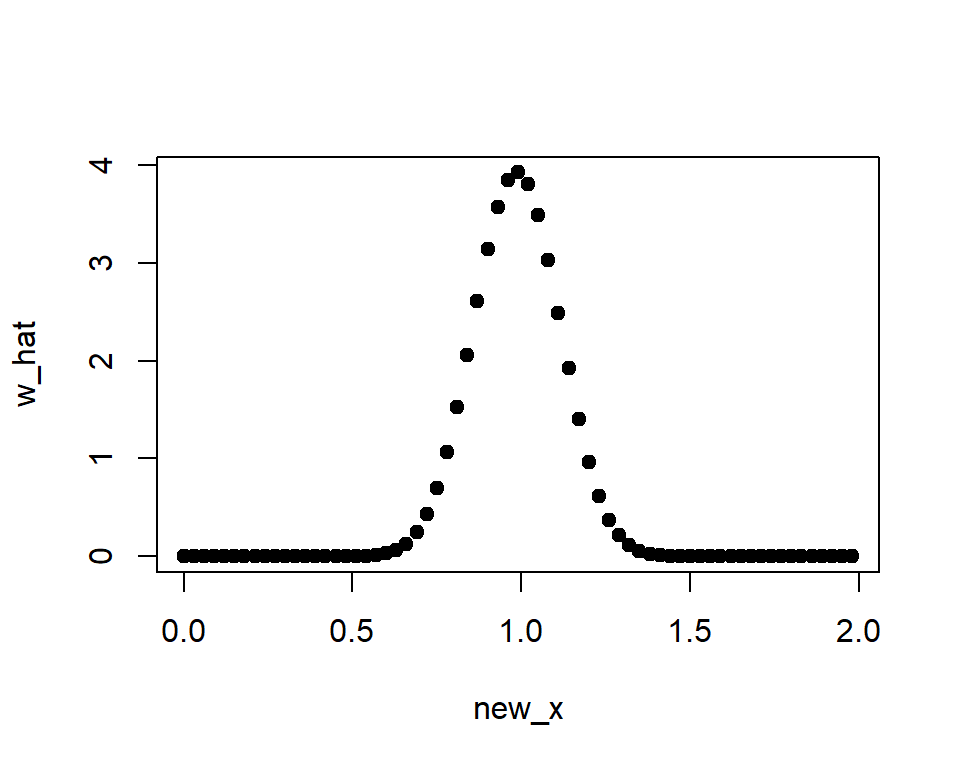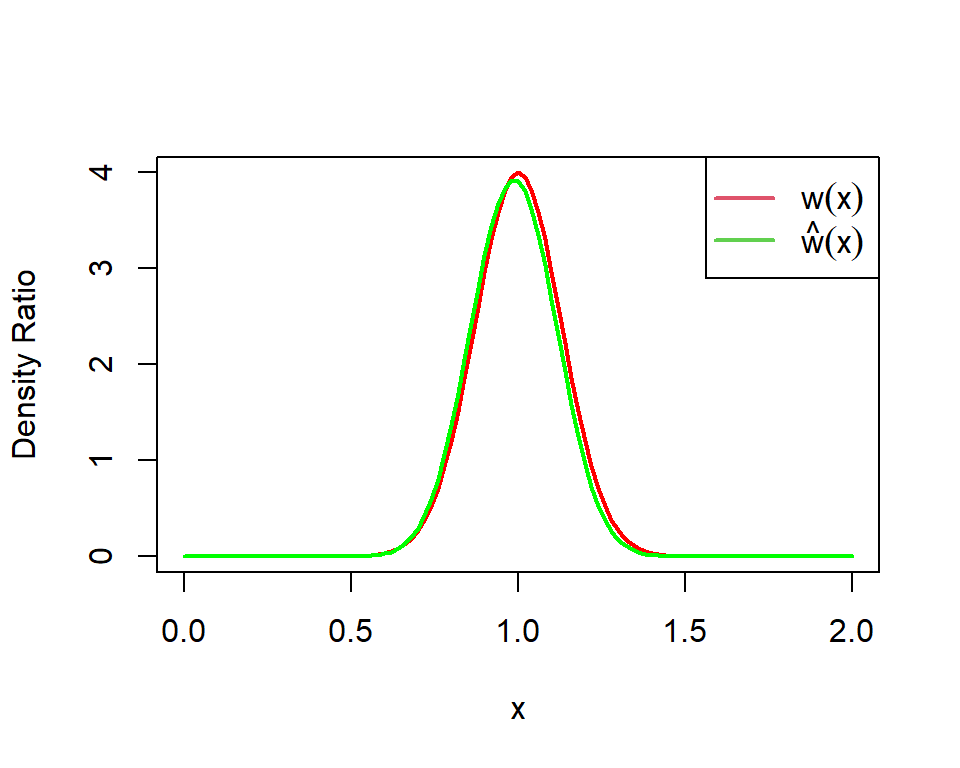# Density Ratio Estimation

Density ratio estimation. The estimated density ratio function can be used in many applications such as anomaly detection, change-point detection, covariate shift adaptation. The implemented methods are uLSIF (Hido et al. (2011) ), RuLSIF (Yamada et al. (2011) ), and KLIEP (Sugiyama et al. (2007) ).

## title: "An R Package for Density Ratio Estimation" author: Koji MAKIYAMA (@hoxo_m) output: html_document: keep_md: true md_document: variant: gfm

Density ratio estimation is described as follows: for given two data samples `x` and `y` from unknown distributions `p(x)` and `q(y)` respectively, estimate `w(x) = p(x) / q(x)`, where `x` and `y` are d-dimensional real numbers.

The estimated density ratio function `w(x)` can be used in many applications such as anomaly detection  and covariate shift adaptation . Other useful applications about density ratio estimation were summarized by Sugiyama et al. (2012) .

The package densratio provides a function `densratio()`. The function outputs an object that has a function to estimate density ratio.

For example,

The function `densratio()` estimates the density ratio of `p(x)` to `q(y)`, `w(x) = p(x)/q(y) = Norm(1, 1/8) / Norm(1, 1/2)`, and provides a function to compute estimated density ratio. The result object has a function `compute_density_ratio()` that can compute the estimated density ratio `w_hat(x)` for any d-dimensional input `x` (now d=1).In this case, the true density ratio `w(x) = p(x)/q(y) = Norm(1, 1/8) / Norm(1, 1/2)` can be computed precisely. So we can compare `w(x)` with the estimated density ratio `w_hat(x)`.## 2. Installation

You can install the densratio package from CRAN.

You can also install the package from GitHub.

The source code for densratio package is available on GitHub at

## 3. Details

`densratio()` has `method` argument that you can pass `"uLSIF"` or `"KLIEP"`.

• uLSIF (unconstrained Least-Squares Importance Fitting) is the default method. This algorithm estimates density ratio by minimizing the squared loss. You can find more information in Hido et al. (2011) .

• KLIEP (Kullback-Leibler Importance Estimation Procedure) is the another method. This algorithm estimates density ratio by minimizing Kullback-Leibler divergence. You can find more information in Sugiyama et al. (2007) .

There is a vignette for the package. For more detail, read it.

You can also find it on CRAN.

## 4. Related work

We have also developed a Python package for density ratio estimation.

The package is available on PyPI (Python Package Index).

## 5. References

 Hido, S., Tsuboi, Y., Kashima, H., Sugiyama, M., & Kanamori, T. Statistical outlier detection using direct density ratio estimation. Knowledge and Information Systems 2011.

 Sugiyama, M., Nakajima, S., Kashima, H., von Bünau, P. & Kawanabe, M. Direct importance estimation with model selection and its application to covariate shift adaptation. NIPS 2007.

 Sugiyama, M., Suzuki, T. & Kanamori, T. Density Ratio Estimation in Machine Learning. Cambridge University Press 2012.

# densratio 0.1.0

## Bug fixes

• Modify Euclidean distance to square Euclidean distance in the function that computes Gaussian kernel (#6, @ya103). It affects all algorithms in the packages.

# Reference manual

install.packages("densratio")

0.2.1 by Koji Makiyama, 2 years ago

https://github.com/hoxo-m/densratio

Report a bug at https://github.com/hoxo-m/densratio/issues

Browse source code at https://github.com/cran/densratio

Authors: Koji Makiyama [aut, cre]

Documentation:   PDF Manual